# How to create and use dynamic named range in Excel

In this tutorial, you will learn how to create a dynamic named range in Excel and how to use it in formulas to have new data included in calculations automatically.

In last week's tutorial, we looked at different ways to define a static named range in Excel. A static name always refers to the same cells, meaning you would have to update the range reference manually whenever you add new or remove existing data.

If you are working with a continuously changing data set, you may want to make your named range dynamic so that it automatically expands to accommodate newly added entries or contracts to exclude removed data. Further on in this tutorial, you will find detailed step-by-step guidance on how to do this.

## How to create a dynamic named range in Excel

For starters, let's build a dynamic named range consisting of a single column and a variable number of rows. To have it done, perform these steps:

1. On the Formula tab, in the Defined Names group, click Define Name. Or, press Ctrl + F3 to open the Excel Name Manger, and click the New… button.
2. Either way, the New Name dialogue box will open, where you specify the following details:
• In the Name box, type the name for your dynamic range.
• In the Scope dropdown, set the name's scope. Workbook (default) is recommended in most cases.
• In the Refers to box, enter either OFFSET COUNTA or INDEX COUNTA formula.
3. Click OK. Done!

In the following screenshot, we define a dynamic named range items that accommodates all cells with data in column A, except for the header row: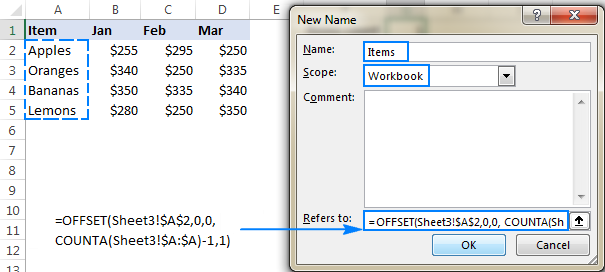### OFFSET formula to define an Excel dynamic named range

The generic formula to make a dynamic named range in Excel is as follows:

OFFSET(first_cell, 0, 0, COUNTA(column), 1)

Where:

• first_cell - the first item to be included in the named range, for example \$A\$2.
• column - an absolute reference to the column like \$A:\$A.

At the core of this formula, you use the COUNTA function to get the number of non-blank cells in the column of interest. That number goes directly to the height argument of the OFFSET(reference, rows, cols, [height], [width]) function telling it how many rows to return.

Beyond that, it's an ordinary Offset formula, where:

• reference is the starting point from which you base the offset (first_cell).
• rows and cols are both 0, since there are no columns or rows to offset.
• width is equal to 1 column.

For example, to build a dynamic named range for column A in Sheet3, beginning in cell A2, we use this formula:

`=OFFSET(Sheet3!\$A\$2, 0, 0, COUNTA(Sheet3!\$A:\$A), 1)`

Note. If you are defining a dynamic range in the current worksheet, you do not need to include the sheet name in the references, Excel will do it for you automatically. If you are building a range for some other sheet, prefix the cell or range reference with the sheet's name followed by the exclamation point (like in the formula example above).

### INDEX formula to make a dynamic named range in Excel

Another way to create an Excel dynamic range is using COUNTA in combination with the INDEX function.

first_cell:INDEX(column,COUNTA(column))

This formula consists of two parts:

• On the left side of the range operator (:), you put the hard-coded starting reference like \$A\$2.
• On the right side, you use the INDEX(array, row_num, [column_num]) function to figure out the ending reference. Here, you supply the entire column A for the array and use COUNTA to get the row number (i.e. the number of non-entry cells in column A).

For our sample dataset (please see the screenshot above), the formula goes as follows:

`=\$A\$2:INDEX(\$A:\$A, COUNTA(\$A:\$A))`

Since there are 5 non-blank cells in column A, including a column header, COUNTA returns 5. Consequently, INDEX returns \$A\$5, which is the last used cell in column A (usually an Index formula returns a value, but the reference operator forces it to return a reference). And because we have set \$A\$2 as the starting point, the final result of the formula is the range \$A\$2:\$A\$5.

To test the newly created dynamic range, you can have COUNTA fetch the items count:

`=COUNTA(Items)`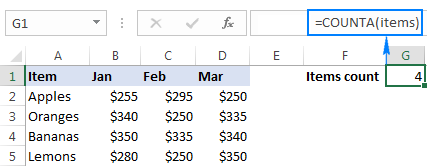If all done properly, the result of the formula will change once you add or remove items to/from the list: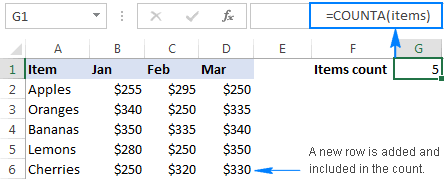Note. The two formulas discussed above produce the same result, however there is a difference in performance you should be aware of. OFFSET is a volatile function that recalculates with every change to a sheet. On powerful modern machines and reasonably sized data sets, this should not be a problem. On low-capacity machines and large data sets, this may slow down your Excel. In that case, you'd better use the INDEX formula to create a dynamic named range.

## How to make two-dimensional dynamic range in Excel

To build a two-dimensional named range, where not only the number of rows but also the number of columns is dynamic, use the following modification of the INDEX COUNTA formula:

first_cell:INDEX(\$1:\$1048576, COUNTA(first_column), COUNTA(first_row)))

In this formula, you have two COUNTA functions to get the last non-empty row and last non-empty column (row_num and column_num arguments of the INDEX function, respectively). In the array argument, you feed the entire worksheet (1048576 rows in Excel 2016 - 2007; 65535 rows in Excel 2003 and lower).

And now, let's define one more dynamic range for our data set: the range named sales that includes sales figures for 3 months (Jan to Mar) and adjusts automatically as you add new items (rows) or months (columns) to the table.

With the sales data beginning in column B, row 2, the formula takes the following shape:

`=\$B\$2:INDEX(\$1:\$1048576,COUNTA(\$B:\$B),COUNTA(\$2:\$2))`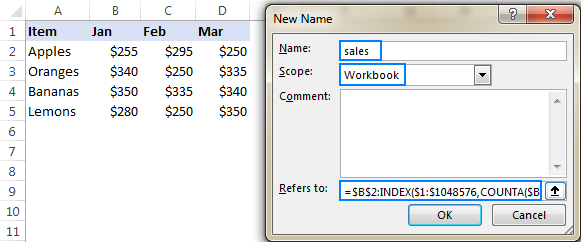To make sure your dynamic range works as it is supposed to, enter the following formulas somewhere on the sheet:

`=SUM(sales)`

`=SUM(B2:D5)`

As you can see in the screenshot bellow, both formulas return the same total. The difference reveals itself in the moment you add new entries to the table: the first formula (with the dynamic named range) will update automatically, whereas the second one will have to be updated manually with each change. That makes a huge difference, uh?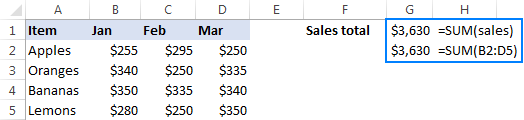## How to use dynamic named ranges in Excel formulas

In the previous sections of this tutorial, you have already seen a couple of simple formulas that use dynamic ranges. Now, let's try to come up with something more meaningful that shows the real value of an Excel dynamic named range.

For this example, we are going to take the classic INDEX MATCH formula that performs Vlookup in Excel:

INDEX (return_range, MATCH (lookup_value, lookup_range, 0))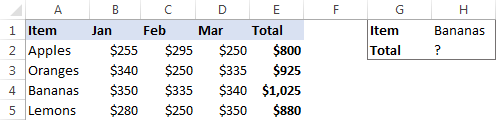…and see how we can make the formula even more powerful with the use of dynamic named ranges.

As shown in the screenshot above, we are attempting to build a dashboard, where the user enters an item name in H1 and gets the total sales for that item in H2. Our sample table created for demonstration purposes contains only 4 items, but in your real-life sheets there can be hundreds and even thousands of rows. Furthermore, new items can be added on a daily basis, so using references is not an option, because you'd have to update the formula over and over again. I'm too lazy for that! :)

To force the formula to expand automatically, we are going to define 3 names: 2 dynamic ranges, and 1 static named cell:

Lookup_range: =\$A\$2:INDEX(\$A:\$A, COUNTA(\$A:\$A))

Return_range: =\$E\$2:INDEX(\$E:\$E, COUNTA(\$E:\$E))

Lookup_value: =\$H\$1

Note. Excel will add the name of the current sheet to all references, so before creating the names be sure to open the sheet with your source data.

Now, start typing the formula in H1. When it comes to the first argument, type a few characters of the name you want to use, and Excel will show all available matching names. Double-click the appropriate name, and Excel will insert it in the formula right away: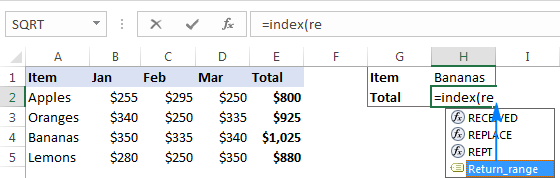The completed formula looks as follows:

`=INDEX(Return_range, MATCH(Lookup_value, Lookup_range, 0))`

And works perfectly!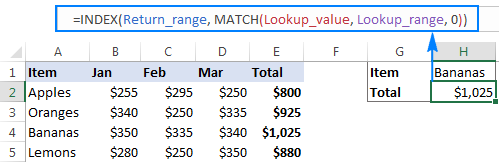As soon as you add new records to the table, they will be included in your calculations at once, without you having to make a single change to the formula! And if you ever need to port the formula to another Excel file, simply create the same names in the destination workbook, copy/paste the formula, and get it working immediately.

Tip. Apart from making formulas more durable, dynamic ranges come in handy for creating dynamic dropdown lists.

This is how you create and use dynamic named ranges in Excel. To have a closer look the formulas discussed in this tutorial, you are welcome to download our sample Excel Dynamic Named Range Workbook. I thank you for reading and hope to see you on our blog next week!

## You may also be interested in

1. Hi -
Not sure if this is relevant --
I want to increment a Cell index number --
eg Cell referenced in sheet -- =Sheet1!Findx
iow how can I modify indx if indx=5 thus F5, how can I modify it with formula to eg F6

use-case -
I have on a spreadsheet a integer in a Cell eg C5 = integer 10
What I want to do --
Extract a value from the Sheet1 -- =Sheet1!(Findx) where the indx will now be the value of integer in C5
iow =Sheet1!F10

How Can I modify the formula such that value retrieved will be =Sheet1F10+offsett
so that if offset is 5 -- The value in Sheet1 @ F15 (10+5) will be retrieved

Thank you

2. Hello. Is it possible to store the values of a dynamic array in a named range already calculated? For example, when I enter this formula for a new named range, the name manager shows the values as an ellipsis:

=ABS(INDEX(A\$1:A\$1000,0,1))

I'm assuming this means Excel will recalculate that formula each time the name is referenced. Is there a way to avoid this without first having to create a column with the absolute values already calculated, and then pointing the new name to that column?

Thank you.

• Hi! If I understand the question correctly, such a formula can work:

=ABS(namedrange)
or
=ABS(INDEX(namedrange,0,1))

3. I'm trying to add a formula in the cell following a sequence formula that is dynamic. The sequence formula can autofill in 6 cells (I want to add the formula in the 7th cell) of the sequence formula can autofill in 8 cells (I want to add the formula in the 9th cell).
Is there a way to do that automatically?

• Hi! You cannot automatically add a formula to a cell.

4. I'm trying to do a graph to display the last 6 days worth of data using OFFSET. The formula works if I have 6 or more days in my table, but I'm receiving reference errors if I have less than 5 days.
E9=01Jan2023
F9=02Jan2023
G9=03Jan2023
H9=04Jan2023 .....

this is my formula.
=OFFSET(\$E\$9,,IF(COUNTA(\$E\$9:\$GA\$9)<6,0,COUNTA(\$E\$9:\$GA\$9)-6),,IF(COUNTA(\$E\$9:\$GA\$9)<6,COUNTA(\$E\$9:\$GA\$9),6))

would you be able to identify where my problem lies or how I can display the graph with only 1, 2, 3, 4 or 5 days worth of data without error message?

• I tested your formula with three dates in cells E9:G9 and got no error.

5. Hi,

I have production report with two columns one column for dates and second column is for case number so I need result how many case number completes for the date.
Example.
A column B column
03/13/23 AS123
03/13/23 AS234
03/14/23 AS768

I need countifs formula for with match date on second sheet.

6. Image attached is the best way to understand what the formula supposed to achieve and the problem I am trying to resolve. However, I will try to explain the requirement which the calculation is trying to satisfy.

Background:

Business Entity (Corporation, such as Amazon) - US Tax Code allow a business to carryforward Operating Loss of a business entity to be used to OFFSET income in future years, until the loss is fully used. Depending on the income in future years, previous year(s) loss can be completely utilized in "One Single Year", or spread across multiple years (as shown in Row 33 - Year = 2017)

As shown in the table below, the first three columns capture the details such as NOL Generated Amount, Used, or Expired for the Year of NOL Generated which is mentioned in column "Year". Column H calculate how much of the income from the Year (mentioned in Year column) is used in "Current Year" (which in this example is 2022 - bottom row).

Now, based on the above-mentioned facts so far, the calculation is straightforward, where Total Available column give me the amount of NOL that can be used for Current Year, and the amount utilized needs to be deducted from the Income as calculation progress through subsequent years.

**
Here comes the Wrinkle and hence my problem, because of Tax Rule Changes:

Post 2017, there is difference between how the NOL is treated (or for that matter, how much of it can be used). NOLs generated prior to 2018 (Year <2018 in column "Year") vs NOLs generated AFTER 2017.

Tax code now limit the amount of NOL taxpayer can use if it was generated after 2017, and the way it does is taking 80% of the Taxable Income left after deducting total Pre-2018 NOL from the Taxable Income.

Example:
Taxable Income = \$1,000,000
Total Pre-2018 NOL (Sum of Column H for Years 2001 through 2017) = \$400,000

Taxable Income AFTER Pre-2018 NOL = \$600,000
NOL Limitation %= 80%
Taxable Income Limit for Post-2017 NOL = \$480,000

Total Post-2017 NOL (Sum of Column H for Years 2018 through 2021) = \$700,000

Taxable Income after NOL = \$120,000
--As you can see, Total Post-2017 NOL = \$700,000 and prior to change in tax rules, entire income would have been OFFSET and still \$100,000 worth of loss was left which can be carried forward into the future, but due to tax rule change, taxpayer can use ONLY \$480,000 of NOL from \$700,000.

**
In the formula I am working on, I need to incorporate above mentioned calculation based on the Year NOL is generated in a "GENERIC" formula, so that I do not have to manually adjust the worksheet every year into the future.

The highlighted cell reference in the expression shown in the image needs to be generated dynamically based on the value in Year column, so that when the formula is dragged down and reaches rows corresponding to Year = 2018, then first part of the SUM range is set as absolute cell reference and the second part is same for 2018 and increases for each subsequent year.

Not able to insert image; hence, manually providing the formulas:

Columns = Year | Total NOL Generated | Previously Utilized | Expired | Total NOL Available | Current Year Utilization | Carryforward | Expiration

Rows:
F12 = Income before NOL
G17 = First Row [Total Available]

Row 1 (2001) = MAX(0,MIN(F12,G17))

Row 2 through 17 (2002 - 2017) = IF(C18<2018,MAX(0,MIN(G18,\$F\$12-SUM(\$H\$17:H17)))

Row 18 (2018) = IF(C35=2018,MAX(0,MIN(G35,((\$F\$12-SUM(\$H\$17:\$H\$33))*\$J\$14)))

Row 19 - 21 (2019 - 2021) = MAX(0,MIN(G35,((\$F\$12-SUM(\$H\$17:\$H\$33))*\$J\$14)-SUM(\$H\$34:H34)))))

**Row 2 through 21 have a generic formula which is combination of all the individual expressions mentioned above. Above the expression is broken down to illustrate which part of the expression condition apply to which rows in the NOL schedule.

The problem is with expression "SUM(\$H\$34:H34)" because as the formula is dragged down from first row with Year = 2018 upwards, the formula falls apart. At this point, I have to manually manage the formula based on where the Year = 2018, and will have to do the same next year, unless the cell reference can be generated dynamically based on value in Year column.

Any suggestion?

7. Good day, I need some assistance please...I have an excel sheet with Dynamic columns. I display the total Working hours in columnD and only display the overtime hours if there are any. If there isn't any overtime hours then I display the week in days starting from either column E or columnG. I need to sort the days of the week according to a date range which I have just specified. So, If my Monday falls on the 19th and my Thursday falls on the 13th, I need to sort the columns so that Thursday comes first and Wednesday comes last. I don't know the column letter (could be D or E or F or G or so on) but I can determine the letter. I don't want to use the letter but when I substitute, I get an error. When I run the hardcoded substitution, it works fine "Sheets(sheetName).Range("I2:O132").Sort Key1:=Range("I2:O2"), Order1:=xlAscending, Orientation:=xlLeftToRight". When I use the following, I get an error Sheets(sheetName).Range(("""" & strColumnStart & "" & ":" & "" & strColumnEnd & """")).Sort Key1:=Range("""" & strColumnStart & "" & ":" & "" & strColumn & """"), Order1:=xlAscending, Orientation:=xlLeftToRight. When I use the immediate window, """" & strColumnStart & "" & ":" & "" & strColumnEnd & """" equates to "I2:O132" but it just doesn't work. Does anyone have a solution to this?

• Hi!
Unfortunately, we can only help with writing Excel formulas. Your problem cannot be solved with formulas.

8. How to Create a name manager if the is many blank cells in between cells

9. =SUMPRODUCT(((Airport!\$B\$5:\$B\$2000)=\$A\$6)*((Airport!\$C\$5:\$C\$2000)=C2),(Airport!D5:D2000)) works perfect so added your formula to create a dynamic range.
=SUMPRODUCT(((Mon_Col2)=\$A\$6)*((Airport!\$C\$5:\$C\$2000)=C2),(Airport!D5:D2000)) when you step in and evaluate the formula it does return the correct dynamic range. However it gives me a #N/A error when finished.

• Hello!
I can't see your data, but I can assume that your dynamic ranges are different sizes.

10. HI,
I have created a table using dynamic range. I would like to format(meaning color/borders) like a when I create a table with alternating fill colors for row. Is there a simple method?
The button Labeled "Format as Table" creates a table them my Dynamic range goes to Spill Error...

Thanks,
Jmarc

11. Here's a weird one for dynamic range defined in Formulas>Name Manager (Excel MAc v16.59)...
Trying to define/name a dynamic range \$L\$1:\$M\$M from Parameters! Tab using:
=OFFSET(Parameters!\$L\$1,0,0,COUNTA(Parameters!\$M:\$M),2)

1) Type that into any cell in the workbook (even other Tabs) and seems to return exactly whats desired...
2) Type that into the Name Manager to assign a name to this dynamic range and get nothing returned... no error message, just an empty set of data.

Any ideas or possibly is there a bug in the Excel Mac?
Thanks for any response...

• Hello!
Unfortunately, I was unable to reproduce your situation on Excel for Mac. I pasted your formula into the "Refers to" field and got a working named range.
Check if you are doing everything correctly in accordance with the instructions described in the article above.

12. I'm having trouble entering the formula for a dynamic range.
or
Keeps giving me a message about am I trying to enter a formula, must start with= or -.
When I enter only the cell formula, it takes it, but I need a range.
This creates dynamic range starting at today's date in column P and I want to extend it to the end of the data in column P. Column P is a list of dates.
I need this to then find the next value in a different column after the today's date row.
This different than most dynamic ranges that only extend the bottom. I want to do both. One to move the top based on today's date and the bottom to extend it based on data being added.
I hope you can help me. I've learned a lot from you.
Thanks,
Colt

• Hello!
If your list of dates starts in cell A1, then you can use the formula to create a dynamic range starting from the current date:

=OFFSET(A1,MATCH(TODAY(),A1:A300,0)-1,0, COUNTA(A1:A300)-MATCH(TODAY(),A1:A300,0)+1,1)

13. Hi,
How can I get the Defined Name to work for a dynamic range when I have both Blank and Non-Blank cells within my range?

• Hello!
Try using the COUNTBLANK function in your dynamic range formula:

OFFSET(first_cell, 0, 0, COUNTA(column)+COUNTBLANK(column), 1)

• I don't think this will work. The COUNTBLANK function will also count all of the blanks below the last item in the column giving you a very large value for modern versions of Excel.

• Hi!
Of course, you can use a range of cells instead of the entire column in the formula.

For example - Instead of COUNTA(column) use COUNTA(A1:A100)

This will seriously speed up the calculations.

14. Each day I copy a watchlist of share prices from Yahoo Finance to Excel. By placing the latest end-of day closing share prices in the right-most column of a table, all the previous entries move one column to the left. (Excel does that for me).
Every day's close-price of each company, from start date to the present, is listed in a row. The column holding the start date, is moving leftwards into another column, day by day.

I make charts of each company. The chart includes the Start date, (when I first started taking the company's data). The chart records up to the present day.

I know where is each start date. By using Index and Match I can find exactly in which column is the Start date. I can list the column reference in a cell:- e.g in cell Q1 is listed, column "CME".

Today, the column holding my start date for the company, RCP.L, is CME. Yesterday, before I took the day's figures, it was CMF.

I now want an automatic facility (formula) to enter CME14:CME770 into an INDEX & MATCH formula (below) which, yesterday was reading CMF14:CMF770:-

So the Index Match formula for yesterday is:-
INDEX(Close!CMF\$14:CMF\$770,MATCH(\$Q\$1,Close!\$ATK\$14:\$ATK\$770,0))
It reads yesterday's range, not today's.

(\$SQ\$S1 reads the cell containing "RCP.L" to find the row in the column range CMF14:CMF770.)

Today I want the INDEX Match formula to be
INDEX(Close!CME\$14:CME\$770,MATCH(\$Q\$1,Close!\$ATK\$14:\$ATK\$770,0))

Tomorrow I will want CMD14:CMD770 in the formula and so-on.

15. Hello!
How do I create a Dynamic Named Range for unlocked ranges by a password when sheet is protected.

16. How do I create a Dynamic Named Range for unlocked range by a password?

17. I would like to add numbers in a column
using sum, offset, counta and the column has a name.
For practice, I have column E and beginning with row 3 the title: salary. Rows 4, 5, 6,etc are the salaries. Column E3:E10 has been named as "monthly_salaries". I need to use that so I can place the sum(monthly_salaries) function on any worksheet, while the monthly-salaries will be on a seperate worksheet.
Each month the length of this vertical column E changes as more or less people draw salaries. Also, I use different worksheets so data, like the salaries may be located on worksheet 2 and the actual sum on worksheet 1.
I have offset(e3,0,0,counta(E:E),1.
Question: How to combine the column name with the sum function to accommodate the dynamic nature of this problem.
Chris

• Hello!
Create a dynamic named range “monthly_salaries” using a formula

=OFFSET(\$E\$3, 0, 0, COUNTA(\$E\$3:\$E\$10), 1)

Use it like this:

=SUM(monthly_salaries)

18. Dynamic Named Ranges don't seem to like Dynamic Array Formulas it seems.
I have 2 Dynamic Named Ranges, say LIST1 and LIST2. In a separate column, I have entered the formula: FILTER(LIST1, LIST2="an existing value"). This returns an error (#VALUE!). It worked correctly when my named ranges were not dynamic.
Is there an evident reason why I am getting this error?

Sabrina

• Hello!
I was unable to repeat your errors. I don't have your data.

19. Hi, How do i use Dynamic Named Range in "Data Rane" Excel chart ?
=\$B\$2:INDEX(\$1:\$1048576,COUNTA(\$B:\$B),COUNTA(\$2:\$2)) or =\$A\$2:INDEX(\$A:\$A, COUNTA(\$A:\$A))
is not suitable for this.
Thank You,
Ofer

20. hi there.. hope someone could help me. I want to create a name in my file which is getting values from a single column table. I want to exclude one specific value and don't want it to be part of the name range. Not sure how can I do that? Column data is:
Apple
Banana
Orange
Grapes
Want to have a list that exclude "Orange". As it's a table I will be adding new names after Grapes in near future.

• Pls refer to: FILTER(A1:INDEX(A:A,COUNTA(A:A)),A1:INDEX(A:A,COUNTA(A:A))"Orange") in the New Name dialog

21. Hello, I am painfully new to working with Excel, macros, and the like. I have recorded a macro, but right now it will only work on the cell range that I originally recorded the macro on. Right now the range reads as =Range("A2:A724"), but I need the bottom part of the range (A724) to be dependent on column B. Meaning, if column B has data going down to cell B1021, then I want my range in column A to automatically look like =Range("A2:A1021"). I would appreciate any help. Thanks!

22. Hi! I'm a newbie in Excel, so there's a risk my question is kind os obvious. I'm trying to connect a Pivot Table in Excel to a Word File, for this, I have to edit the Name of my range in Excel and Word so the Word table automatically updates the range it is reporting. The problem I have is Excel says he doesn't understand my formula, it looks like this:

='DE40'!\$B\$4:\$B\$4:INDEX('DE40'!\$1:\$1048576,COUNTA('DE40'!\$B:\$B),+'DE40'!\$4:\$4))

Where DE40 is the Sheet/Pivot Table I'm trying to refer. Any ideas?

23. I have a simple table: 7 columns, 4 rows. Can I create a formula just adding Hrs of bananas?

Jan 1 Jan 2 Jan 3
Hrs Cases Hrs Cases Hrs Cases
Bananas 1 10 3 30 0 0
Apples 5 20 1 4 3 12

• _______|___Hrs_|_Cases_|_Hrs_|_Cases_|_Hrs_|_Cases
Bananas|____1__|___10__|__3__|___30___|_0__|___0__
Apples_|____5__|___20__|__1__|___04___|_3__|___12_

No sure if this will help to understand the table...

24. An easy way to do it if you need a range is to use indirect in the name.. eg example for A2:G10
There is 20 rows in coloumn A

=Indirect(Sheet_name!A2:G"&CountA(A:A))

since indirect "translate" the expression - so it reads the name to be refered to this
Sheet_name!A2:G10

• Correction - There is 20 rows in coloumn A - should ofcourse be 10 rows ;)

25. I'm trying to create a dynamic range showing client names only if their status is Active in another column. Can someone help me with the formula for this?

• Pls refer to: FILTER(A1:INDEX(A:A,COUNTA(A:A)),B1:INDEX(B:B,COUNTA(B:B))="Active") in the New Name dialog

26. Hi,

This index formula method for creating dynamic named range is not working.

Sheet3!\$H\$2:INDEX(Sheet3!\$H:\$H,COUNTA(Sheet3!\$H:\$H))

Using evaluate formula, this shows to result to \$H\$2:\$H\$6. But post that it acts as an array formula. Its finally returning value either H2/H3/H4/H5/H6 based on the cell.
What i mean to say is, it is not giving a range. Hence, this seems wrong.

27. Hi
How do you create a Dynamic named range (with INDEX) but without the sheet name automatically added after the Define Name editor is closed?
Tnks

28. THANK YOU FOR ENLIGHTENING ME
AM SO DAMN GRATEFUL FOR THIS WRITE UP

29. Thank you

30. How do I create a Dynamic Named Range with worksheets instead of rows or columns?

I have a workbook that I add a new worksheet to every week and I want to track my vacation time over the course of the calendar year.